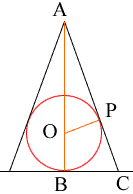Quandaries and Queries Question: A sphere with radius 5cm is inscribed in a right circular cone 20 cm in height.find (a) the base radius ,volume of the cone (b)volume of the shaded space( to 3 sig fig) Hi, I drew a diagram and labeled some points.Angle ABC is a right angle and since AC is tangent to the circle, angle OPA is also a right angle. Thus triangles AOP and ABC are similar. Now list some facts that you know. |AB| = 20 cm |OB| = |OP| = 5 cm thus |AO| = 15 cm From Pythagoras' theorem AP = sqrt(|AO|2 - |OP|2) = 10 sqrt(2) From the similar triangles |OA|/|AC| = |AP|/|AB| and |OA|/|AC| = |OP|/|BC| Find |BC| Penny Go to Math Central